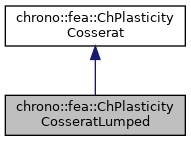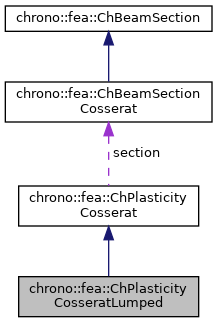chrono::fea::ChPlasticityCosseratLumped Class Reference

## Description

Lumped plasticity of Cosserat-type beams.

This defines 6 independent yelds for the six generalized forces/moments in the beam. Note that this is a rough approximation of plasticity in beams for at least two main reasons: it cannot capture how plastic zones are made inside a section (which is mostly important when cycling with back and forth bending), and it does not capture coupled My+Mz effects, or Nx+My or Nx+Mz as often happens. Briefly: use it if plasticization happens in a scenario of pure bending on a single xy or xz plane, or pure compression/extension, or pure torsion. This can be shared between multiple beams.

#include <ChBeamSectionCosserat.h>

Inheritance diagram for chrono::fea::ChPlasticityCosseratLumped:[legend]
Collaboration diagram for chrono::fea::ChPlasticityCosseratLumped:[legend]

## Public Member Functions

ChPlasticityCosseratLumped ()
Default constructor: linear isotropic constant hardening.

virtual bool ComputeStressWithReturnMapping (ChVector<> &stress_n, ChVector<> &stress_m, ChVector<> &e_strain_e_new, ChVector<> &e_strain_k_new, ChBeamMaterialInternalData &data_new, const ChVector<> &tot_strain_e, const ChVector<> &tot_strain_k, const ChBeamMaterialInternalData &data) override
Given a trial strain, it computes the effective stress and strain by clamping against the yeld surface. More...

virtual void CreatePlasticityData (int numpoints, std::vector< std::unique_ptr< ChBeamMaterialInternalData >> &plastic_data) override

virtual void SetAsRectangularSection (double width_y, double width_z) override
Shortcut: set parameters at once, given the y and z widths of the beam assumed with rectangular shape. More...

virtual void SetAsCircularSection (double diameter) override
Shortcut: set parameters at once, given the diameter of the beam assumed with circular shape. More...Public Member Functions inherited from chrono::fea::ChPlasticityCosserat
virtual void ComputeStiffnessMatrixElastoplastic (ChMatrixDynamic<> &K, const ChVector<> &strain_e, const ChVector<> &strain_k, const ChBeamMaterialInternalData &data)
Compute the 6x6 tangent material stiffness matrix [Km]=d/d, given actual internal data and deformation and curvature (if needed). More...

## Public Attributes

std::shared_ptr< ChFunctionn_yeld_x
sigma_y(p_strain_acc)

std::shared_ptr< ChFunctionn_beta_x
beta(p_strain_acc)

std::shared_ptr< ChFunctionn_yeld_y
sigma_y(p_strain_acc)

std::shared_ptr< ChFunctionn_beta_y
beta(p_strain_acc)

std::shared_ptr< ChFunctionn_yeld_z
sigma_y(p_strain_acc)

std::shared_ptr< ChFunctionn_beta_z
beta(p_strain_acc)

std::shared_ptr< ChFunctionn_yeld_Mx
sigma_y(p_strain_acc)

std::shared_ptr< ChFunctionn_beta_Mx
beta(p_strain_acc)

std::shared_ptr< ChFunctionn_yeld_My
sigma_y(p_strain_acc)

std::shared_ptr< ChFunctionn_beta_My
beta(p_strain_acc)

std::shared_ptr< ChFunctionn_yeld_Mz
sigma_y(p_strain_acc)

std::shared_ptr< ChFunctionn_beta_Mz
beta(p_strain_acc)Public Attributes inherited from chrono::fea::ChPlasticityCosserat
ChBeamSectionCosseratsection

double nr_yeld_tolerance

int nr_yeld_maxiters

## Member Function Documentation

 bool chrono::fea::ChPlasticityCosseratLumped::ComputeStressWithReturnMapping ( ChVector<> & stress_n, ChVector<> & stress_m, ChVector<> & e_strain_e_new, ChVector<> & e_strain_k_new, ChBeamMaterialInternalData & data_new, const ChVector<> & tot_strain_e, const ChVector<> & tot_strain_k, const ChBeamMaterialInternalData & data )
overridevirtual

Given a trial strain, it computes the effective stress and strain by clamping against the yeld surface.

An implicit return mapping integration step is computed automatically per each call of this function. Returns true if it had to do return mapping, false if it was in elastic regime

<<<< sigma_y(p_strain_acc)

<<<< beta(p_strain_e)

<<<< sigma_y(p_strain_acc)

<<<< beta(p_strain_acc)

<<<< sigma_y(p_strain_acc)

<<<< beta(p_strain_acc)

<<<< sigma_y(p_strain_acc)

<<<< beta(p_strain_acc)

<<<< sigma_y(p_strain_acc)

<<<< beta(p_strain_acc)

<<<< sigma_y(p_strain_acc)

<<<< beta(p_strain_acc)

<<<< sigma_y(p_strain_acc)

<<<< beta(p_strain_acc)

Parameters
 stress_n local stress (generalized force), x component = traction along beam stress_m local stress (generalized torque), x component = torsion torque along beam e_strain_e_new updated elastic strain (deformation part) e_strain_k_new updated elastic strain (curvature part) data_new updated material internal variables, at this point, including {p_strain_e, p_strain_k, p_strain_acc} tot_strain_e trial tot strain (deformation part): x= elongation, y and z are shear tot_strain_k trial tot strain (curvature part), x= torsion, y and z are line curvatures data current material internal variables, at this point, including {p_strain_e, p_strain_k, p_strain_acc}

Implements chrono::fea::ChPlasticityCosserat.

 virtual void chrono::fea::ChPlasticityCosseratLumped::SetAsCircularSection ( double diameter )
overridevirtual

Shortcut: set parameters at once, given the diameter of the beam assumed with circular shape.

Implements chrono::fea::ChPlasticityCosserat.

 virtual void chrono::fea::ChPlasticityCosseratLumped::SetAsRectangularSection ( double width_y, double width_z )
overridevirtual

Shortcut: set parameters at once, given the y and z widths of the beam assumed with rectangular shape.

Implements chrono::fea::ChPlasticityCosserat.